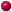# POLIP

##robustFlows-cap1-A

 Name robustFlows-cap1-A Classification nc|cc|d2 Problem type robustflows Description Bilinear problem testing whether a flow problem is robust against a set of demand vectors - instance robustFlows-cap1-A Objective sense min Variables 22033  (0 binary, 0 general integer, 22033 continuous) Nonlinear variables 22033 Constraints 12001 Nonlinear constraints 1 Linear nonzeros 43000 Nonlinear nonzeros 11001 Download robustFlows-cap1-A.pip.gz robustFlows-cap1-A.zpl.gz Best known solution Best known objective Best known bound Originator Lea Rausch, Marc Pfetsch Formulator Lea Rausch, Marc Pfetsch Donator Marc Pfetsch References Minoux10 Links netgen Additional information This is a bilinear model dealing with the following problem. Given a minimum cost flow problem with demand/supply nodes and a demand/supply vector polytope D, can every demand/supply vector in D be transported through the network? Minoux  showed that this problem is NP-hard. The problem can be formulated as a bilinear problem as follows. Suppose that A is the node-arc incidence matrix, and let l and u be lower and upper bounds on the flow x, respectively. Then the original problem is: Does there exist a flow vector x with Ax = d, l ≤ x ≤ u for every d ∈ D? If for some d ∈ D, there does not exist such a flow, by the Farkas lemma, there exist vectors y, s, and t such that AT y + s - t = 0, dT y + uT s - lTt < 0, s, t ≥ 0. Hence, one can consider the following bilinear minimization problem: min { dTr + uT s - lTt : AT y + s - t = 0, s, t ≥ 0, d ∈ D }. If its value is < 0, the answer to the original problem is "no" (i.e., there exists some d ∈ D that is not transportable), otherwise the answer is "yes". The flow instances have been generated using netgen. The demand polytopes are given by a box with the original flow as a base point and increasing the flow by 1% and reducing it by 10% for every node. Additionally, we have a balancing equation, i.e., the sum over all components is 0. All instances with "A" at the end correspond to "yes" instances, while "B" refers to "no" instances. The "yes" instances should be hard to solve, since here one has to prove a nonnegative lower bound.

© by maintainers  |     |  imprint